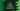# Dart example program to iterate through a list### Introduction :

In this tutorial, we will learn how to iterate through a list of items in dart. List holds items of similar type. The sample program we are going to check here will first create one list of integers, then it will add few integers to the list and finally it will iterate through the list and print out the values. Let’s take a look :

### Using for loop :

Iterating through a list using a loop is the basic way everyone uses. Simply, we will use one for loop that will iterate through the items of the list one by one :

``````main(List args) {
List numbers = new List();

for(var i = 0;i<numbers.length;i++){
print("Position \$i : \${numbers[i]} ");
}
}
``````

So, we have created one list, add few numbers and then iterate through them using a for loop. It will print the below output :

``````Position 0 : 3
Position 1 : 6
Position 2 : 9
Position 3 : 8
Position 4 : 2
Position 5 : 5
Position 6 : 1
``````

The first index of the list starts from 0 and ends on 6.Also we have printed out the numbers one by one.

### Using forEach()

Instead of using a for loop, we can also use forEach() method to iterate through a dart list. Like below :

``````main(List args) {
List numbers = new List();

var i = 0;
numbers.forEach((e) => print("position for \${i++} is \${e}"));
}
``````

Output :

``````position for 0 is 3
position for 1 is 6
position for 2 is 9
position for 3 is 8
position for 4 is 2
position for 5 is 5
position for 6 is 1
``````

### Conclusion :

We have seen two different ways to iterate through a loop in dart. Instead of for loop, you can also use while loop to do the iteration. Try the above examples and drop a comment below if you have any queries.